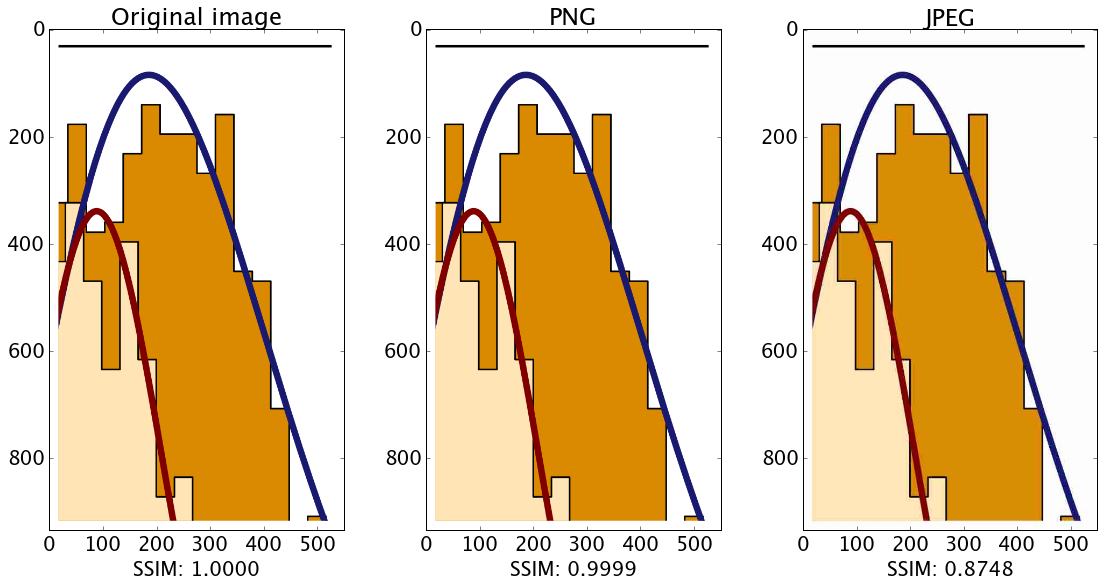Image quality

A ȷupyter notebook

If I would be asked to nominate the one property which characterizes the modern world best, it would be its increasing focus on graphical representations. We are experiencing a transition from a textual to a pictorial society. People communicate with each other not by sending texts, but by photographs on social networks or emojis in chat applications. They learn how to do that not by reading a manual, but by video tutorials. Even highly technical articles on IT oriented websites are invariably illustrated, however unrelated or absurd the respective illustration may be.

I have no serious problem with that as long as the images are a pleasure to look at and are nice to my band width. For keeping this balance, however, a certain understanding of graphic formats is helpful. Only yesterday I received a two-page abstract for a conference which weighed a hefty 73 MB. Saving the images in an appropriate format reduced the size to 1.2 MB. That was not even the most extreme example I've experienced with excessively large files...

More frequently, however, the problem is not an excessive image size but a mediocre image quality. To avoid this problem requires either a certain perceptiveness, or an algorithm suitable for an objective ranking of image quality. The structural similarity index (SSIM) is such an algorithm, and is widely used as video quality metrics in the televison industry.

The present ȷupyter notebook calculates the SSIM for two bitmaps derived from a vector graphics. The first bitmap is obtained by

pdftocairo -png -r 1200 org.pdf org.png
pngquant org.png web.png

The resulting bitmaps measure 550×934 px, and the files 'org.png' and 'web.png' are 30519 and 14797 bytes in size, respectively — significantly larger than the original graphics 'org.pdf' (4214 bytes).

Can we reduce this size further by converting the image to the popular jpeg format? As was to be expected for a line graphics with hard contrasts, we can, but pay the prize of a much reduced image quality. Indeed, to obtain a jpeg file of similar size (to be precise, 15556 bytes) we have to use very low settings:

convert org.png -quality 20 lossy.jpg

As you see in the comparison below, the SSIM for 'web.png' is almost identical to the original, while that of 'lossy.jpg' is drastically lower. And many of you will probably wonder why, since you don't see a difference in the images below – right?

I do, but I have a trained eye. To convince you that the SSIM is meaningful, here are the two images for direct inspection: web.png lossy.jpg.

%matplotlib inline

import numpy as np
import matplotlib as mpl
import matplotlib.pyplot as plt

from skimage import img_as_float
import skimage.io as io
from skimage.measure import compare_ssim as ssim

mpl.rcParams['font.size'] = 20
mpl.rcParams['figure.figsize'] = (16, 8)

img_org = img_as_float(org)
img_png = img_as_float(org_png)
img_jpg = img_as_float(org_jpg)

fig, (ax0, ax1, ax2) = plt.subplots(nrows=1, ncols=3)
plt.tight_layout()

ssim_none = ssim(img_org, img_org, multichannel=True,
dynamic_range=img_org.max() - img_org.min())
ssim_png = ssim(img_org, img_png, multichannel=True,
dynamic_range=img_png.max() - img_png.min())
ssim_jpg = ssim(img_org, img_jpg, multichannel=True,
dynamic_range=img_jpg.max() - img_jpg.min())

label = 'SSIM: %.4f'

ax0.imshow(img_org, cmap=plt.cm.gray, vmin=0, vmax=1)
ax0.set_xlabel(label % (ssim_none))
ax0.set_title('Original image')

ax1.imshow(img_png, cmap=plt.cm.gray, vmin=0, vmax=1)
ax1.set_xlabel(label % (ssim_png))
ax1.set_title('PNG')

ax2.imshow(img_jpg, cmap=plt.cm.gray, vmin=0, vmax=1)
ax2.set_xlabel(label % (ssim_jpg))
ax2.set_title('JPEG')

plt.show()